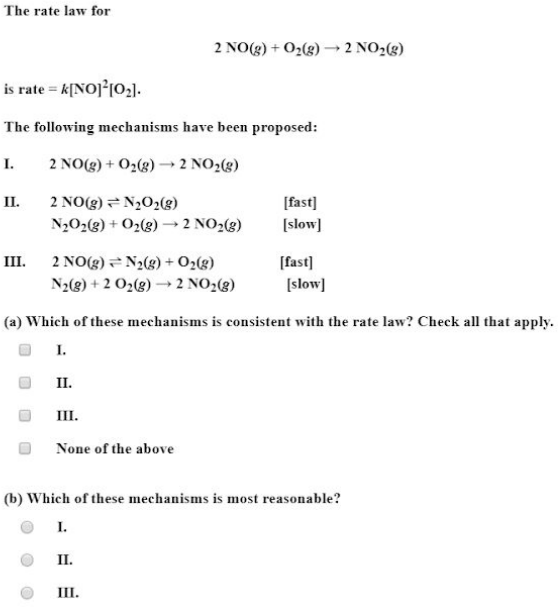# The rate law for 2 NO(g) + O2(g) -> 2 NO2(g) is rate = k[NO]^2[O2]. The following mechanism have been proposed: I. 2 NO(g) + O2(g) -> 2 NO2(g) II. 2 NO(g) <-> N2O2(g) [fast] N2O2(g) + O2(g) -> 2 NO2(g) [slow] III. 2 NO(g) <-> N2(g) + O2(g) [fast] N2(g) + 2 O2(g) -> 2 NO2(g) [slow] (a) Which of these mechanisms is consistent with the rate law? (b) Which of these mechanisms is most reasonable?